# Circle : Equation of Circle , Equation of Circle in different cases , Position of a Point with respect to circle.

Equation of Circle

Let C(i, j) be the centre of the circle and CP(r) be the radius of the Circle, then the equation of the circle is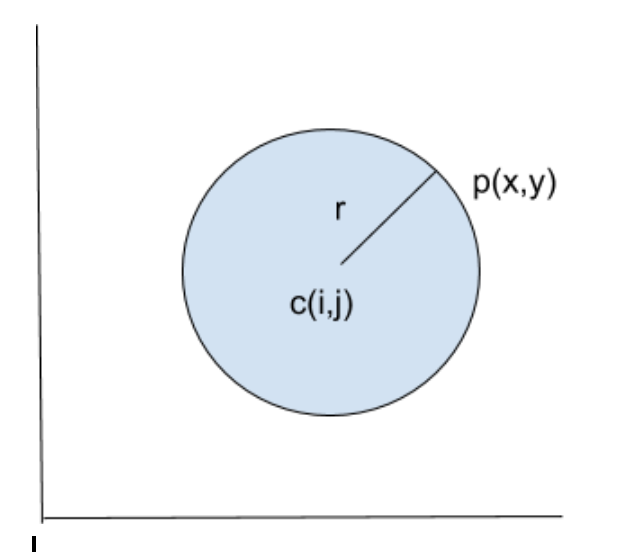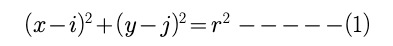Now, origin (0, 0) be the centre of the circle then equation 1 becomes,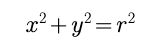The area of the circle is given by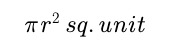General Equation of Circle

The General equation of the second degree may represent a circle if the coeffcient of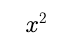and coeffcient of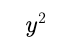are identical and coeffcient of xy becomes zero i.e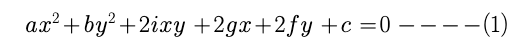represents a circle if a =b i.e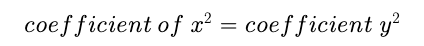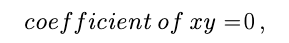then equation 1 reduces as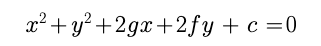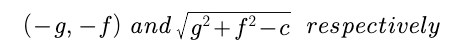Equation of circle in diameter form

Let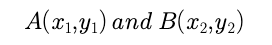be the end points of a diameter of the given circle and let P(x,y) be any point on the circle such that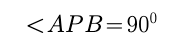Slope of AP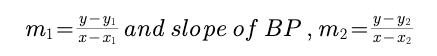for a perpendicular,  m1 * m2 = 1 i.e AP * BP = -1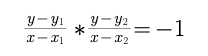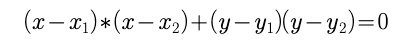Equation of Circle in different cases

Case 1: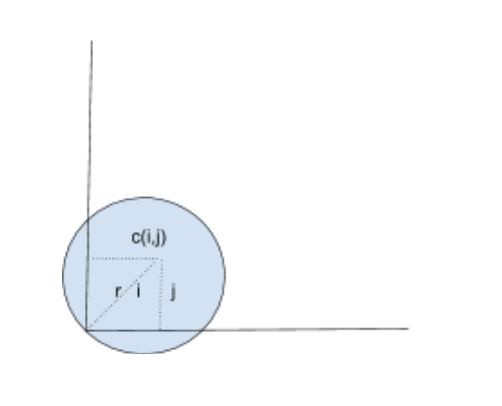When circle passes through origin (0,0). let the equation of the circle beit passes through origin (0,0)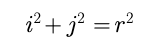equation 1 becomes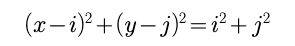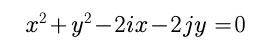Case 2:

When circle passes through x- axis. let the centre of the circle be C(i, j) and toches x-axis at point P , then radius of the circle is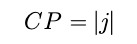equation of the circle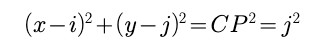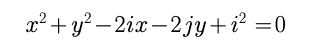Case 3:

When circle passes through y- axis. let the centre of the circle be C(i, j) and toches y-axis at point P , then radius of the circle is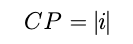equation of the circle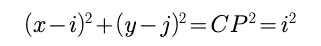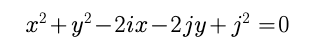Case 4:

When circle toches both axis. In this case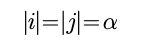then equation of the circle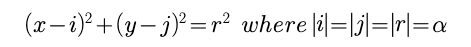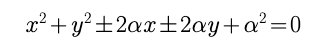Post By : Rahul Kumar 29 Jan, 2020 2829 views Maths